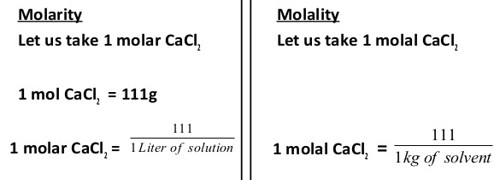# Differences between Molar Solution and Molal Solution

Molarity, also known as molar concentration, is the number of moles of a material per liter of solution. Solutions labeled with the molar concentration are denoted with a capital (M). A 1.0 M solution contains 1 mole of solute per liter of solution.​

E.g., A 1.0 molar solution is a solution that contains one mole of a solute dissolved in a liter of solution. Furthermore, this is a term of concentration, and we call it the “molarity” of the solution. The symbol for this term is “M”. The unit of measurement is mol/L.

Molality is the number of moles of solute per kilogram of solvent. It is vital the mass of solvent is used and not the mass of the solution. Solutions labeled with molal concentration are denoted with a lower case (m). A 1 molal solution is a solution that contains one mole of a solute dissolved in a kilogram of a solution. Hence, the unit of measurement is mol/kg.

Differences between molar solution and molal solution:

Molar solution – It is defined as the number of moles of a solute that are dissolved in 1 liter of a solution.

• Molarity is a concentration of a substance that is calculated as the number of moles of solute that is dissolved in 1 liter of solution. The solution in which one-mole solute remains in one-liter solution is called molar solution.
• Molarity changes with the change of volume of the solution.
• Molarity changes with the change of temperature.
• The unit of the molar solution in mol/liter. The symbol for molarity is (M).
• Molarity is less popular for measuring the concentration of the solution.
• Molarity is affected by changes in pressure.
• Molarity might result in an imprecise and inaccurate concentration. It involves a liquid which means that the concentration can change. This is because the volume being a liquid can change with changes in temperature and pressure.

Example: An aqueous 1.0 molar solution of NaCl (sodium chloride) means a solution of sodium chloride containing one mole of NaCl dissolved in a liter of water.Molal solution – It is defined as the number of moles of a solute that are dissolved in 1 kg of a solvent.

• Molality is a concentration of a substance that is calculated as the number of moles of solute found in 1 kg of solvent. The solution in which one-mole solute remains in 1000 gram solvent is called molal solution.
• Molality changes with the change of mass of the solvent and independent of the volume of solution.
• Molality does not change with the change of temperature.
• The unit of a molal solution is mol/kg. The symbol for molality is (m).
• Molality is more popular for measuring the concentration of the solution.
• Molality is unaffected by changes in pressure.
• Molality results in an accurate precise measurement of concentration. It is a more precise and accurate means of making a certain concentration because it is unaffected by temperature and pressure changes.

Example: A 1 molal solution of sodium chloride means an aqueous solution of NaCl containing one mole NaCl dissolved in a kilogram of water.